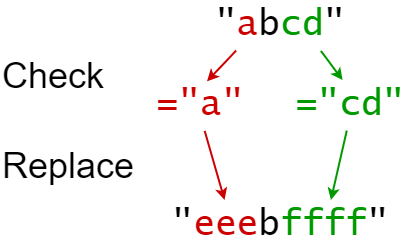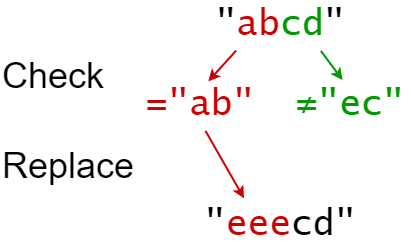# GeetCode Hub

You are given a 0-indexed string `s` that you must perform `k` replacement operations on. The replacement operations are given as three 0-indexed parallel arrays, `indices`, `sources`, and `targets`, all of length `k`.

To complete the `ith` replacement operation:

1. Check if the substring `sources[i]` occurs at index `indices[i]` in the original string `s`.
2. If it does not occur, do nothing.
3. Otherwise if it does occur, replace that substring with `targets[i]`.

For example, if `s = "abcd"`, `indices[i] = 0`, `sources[i] = "ab"`, and `targets[i] = "eee"`, then the result of this replacement will be `"eeecd"`.

All replacement operations must occur simultaneously, meaning the replacement operations should not affect the indexing of each other. The testcases will be generated such that the replacements will not overlap.

• For example, a testcase with `s = "abc"`, `indices = [0, 1]`, and `sources = ["ab","bc"]` will not be generated because the `"ab"` and `"bc"` replacements overlap.

Return the resulting string after performing all replacement operations on `s`.

A substring is a contiguous sequence of characters in a string.

Example 1:```Input: s = "abcd", indices = [0, 2], sources = ["a", "cd"], targets = ["eee", "ffff"]
Output: "eeebffff"
Explanation:
"a" occurs at index 0 in s, so we replace it with "eee".
"cd" occurs at index 2 in s, so we replace it with "ffff".
```

Example 2:```Input: s = "abcd", indices = [0, 2], sources = ["ab","ec"], targets = ["eee","ffff"]
Output: "eeecd"
Explanation:
"ab" occurs at index 0 in s, so we replace it with "eee".
"ec" does not occur at index 2 in s, so we do nothing.
```

Constraints:

• `1 <= s.length <= 1000`
• `k == indices.length == sources.length == targets.length`
• `1 <= k <= 100`
• `0 <= indexes[i] < s.length`
• `1 <= sources[i].length, targets[i].length <= 50`
• `s` consists of only lowercase English letters.
• `sources[i]` and `targets[i]` consist of only lowercase English letters.

class Solution { public: string findReplaceString(string s, vector<int>& indices, vector<string>& sources, vector<string>& targets) { } };